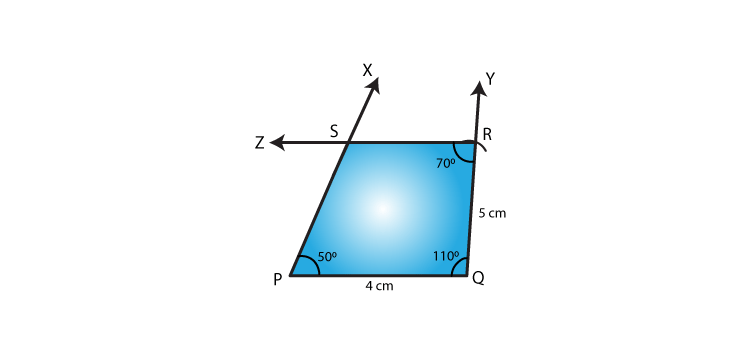# RD Sharma Solutions for Class 8 Maths Chapter 18 Practical Geometry (Constructions) Exercise 18.5

Exercise 18.5 Chapter 18, of RD Sharma, the concept of construction of a quadrilateral when its three angles and their two included sides are given are explained by our tutors with examples. The procedures to construct a quadrilateral are provided here with steps, which is very helpful for students to prepare for their exams. For further assistance, students can refer to RD Sharma Class 8 Solutions formulated by experts at BYJU’S which has all the shortcut methods to solve any question. For better understanding, students can download the pdf from the links provided below.

## Download the Pdf of RD Sharma Solutions for Class 8 Maths Exercise 18.5 Chapter 18 Practical Geometry (Constructions)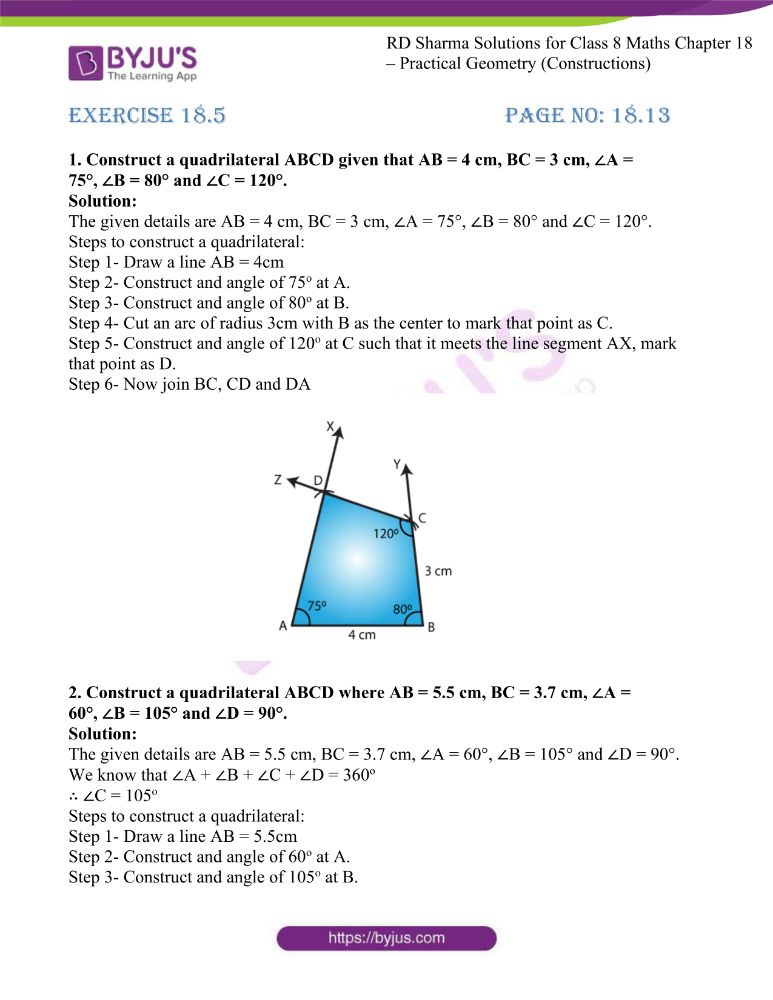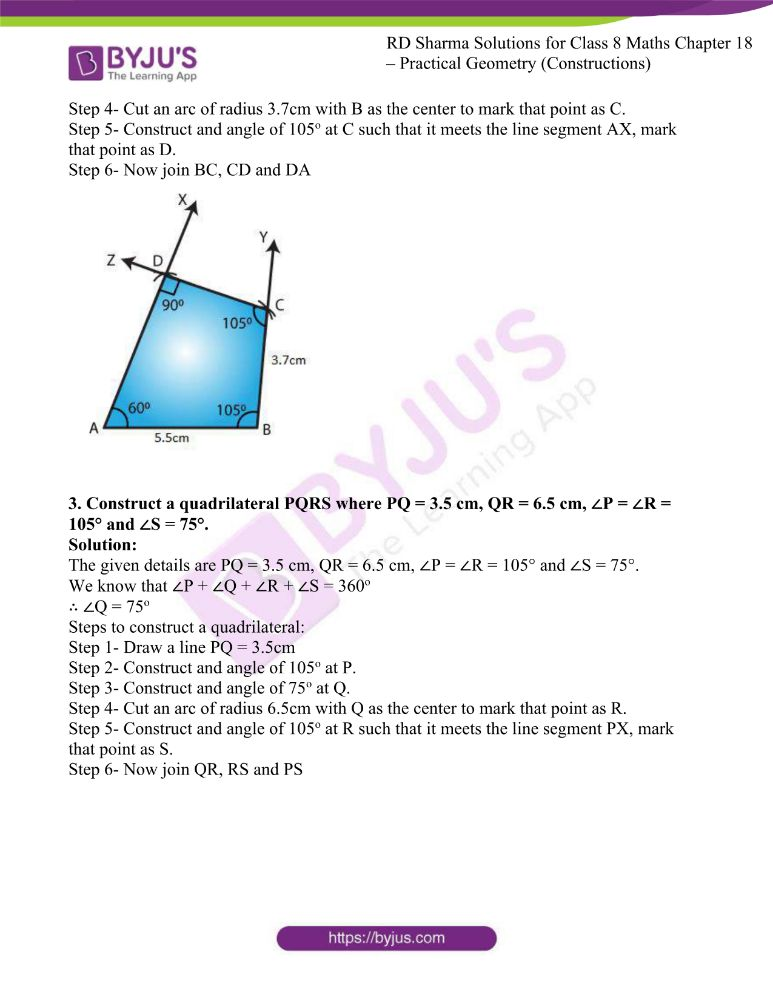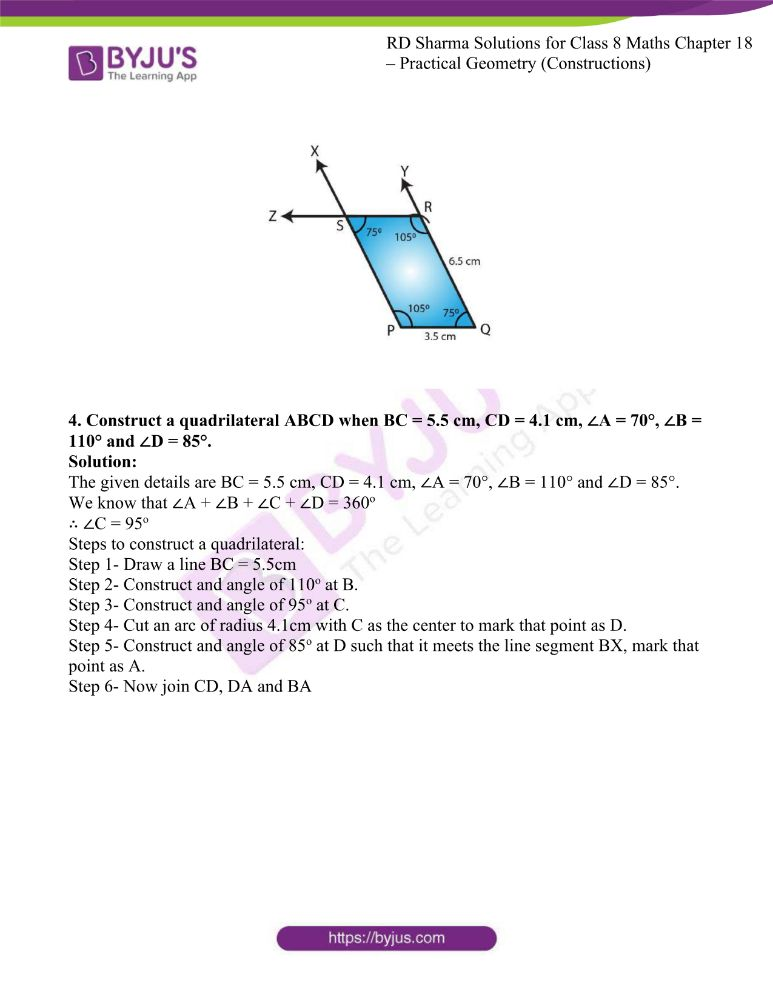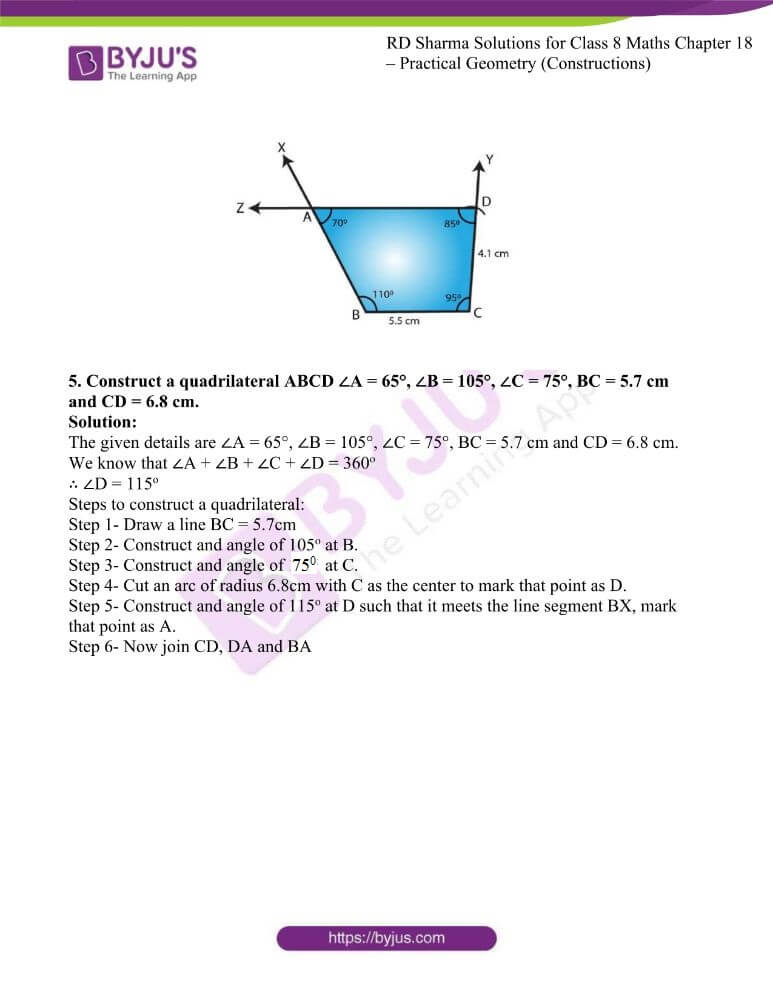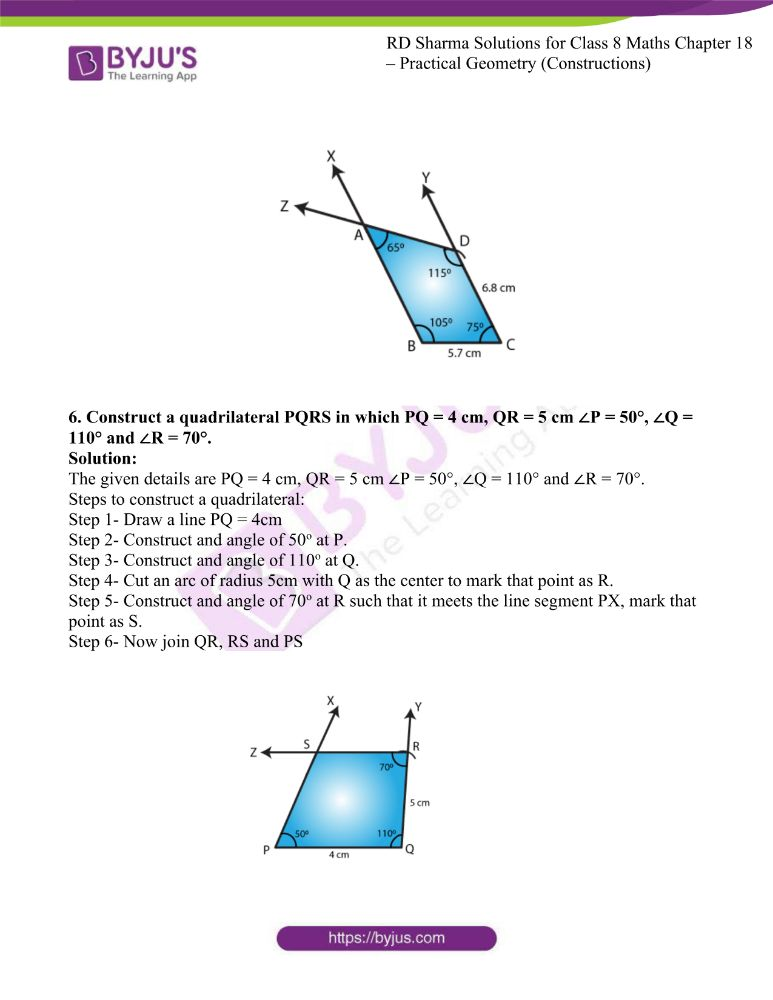### Access answers to RD Sharma Maths Solutions For Class 8 Exercise 18.5 Chapter 18 Practical Geometry (Constructions)

1. Construct a quadrilateral ABCD given that AB = 4 cm, BC = 3 cm, ∠A = 75°, ∠B = 80° and ∠C = 120°.

Solution:

The given details are AB = 4 cm, BC = 3 cm, ∠A = 75°, ∠B = 80° and ∠C = 120°.

Step 1- Draw a line AB = 4cm

Step 2- Construct and angle of 75o at A.

Step 3- Construct and angle of 80o at B.

Step 4- Cut an arc of radius 3cm with B as the center to mark that point as C.

Step 5- Construct and angle of 120o at C such that it meets the line segment AX, mark that point as D.

Step 6- Now join BC, CD and DA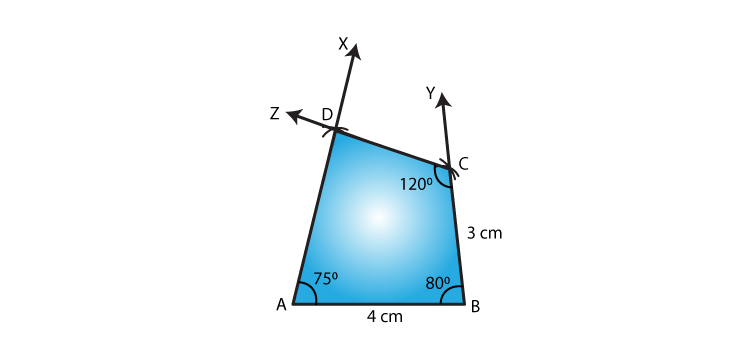2. Construct a quadrilateral ABCD where AB = 5.5 cm, BC = 3.7 cm, ∠A = 60°, ∠B = 105° and ∠D = 90°.

Solution:

The given details are AB = 5.5 cm, BC = 3.7 cm, ∠A = 60°, ∠B = 105° and ∠D = 90°.

We know that ∠A + ∠B + ∠C + ∠D = 360o

∴ ∠C = 105o

Step 1- Draw a line AB = 5.5cm

Step 2- Construct and angle of 60o at A.

Step 3- Construct and angle of 105o at B.

Step 4- Cut an arc of radius 3.7cm with B as the center to mark that point as C.

Step 5- Construct and angle of 105o at C such that it meets the line segment AX, mark that point as D.

Step 6- Now join BC, CD and DA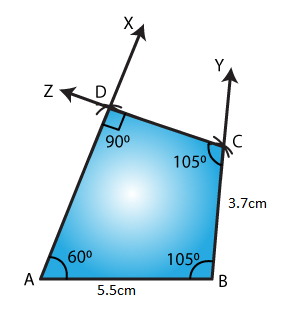3. Construct a quadrilateral PQRS where PQ = 3.5 cm, QR = 6.5 cm, ∠P = ∠R = 105° and ∠S = 75°.

Solution:

The given details are PQ = 3.5 cm, QR = 6.5 cm, ∠P = ∠R = 105° and ∠S = 75°.

We know that ∠P + ∠Q + ∠R + ∠S = 360o

∴ ∠Q = 75o

Step 1- Draw a line PQ = 3.5cm

Step 2- Construct and angle of 105o at P.

Step 3- Construct and angle of 75o at Q.

Step 4- Cut an arc of radius 6.5cm with Q as the center to mark that point as R.

Step 5- Construct and angle of 105o at R such that it meets the line segment PX, mark that point as S.

Step 6- Now join QR, RS and PS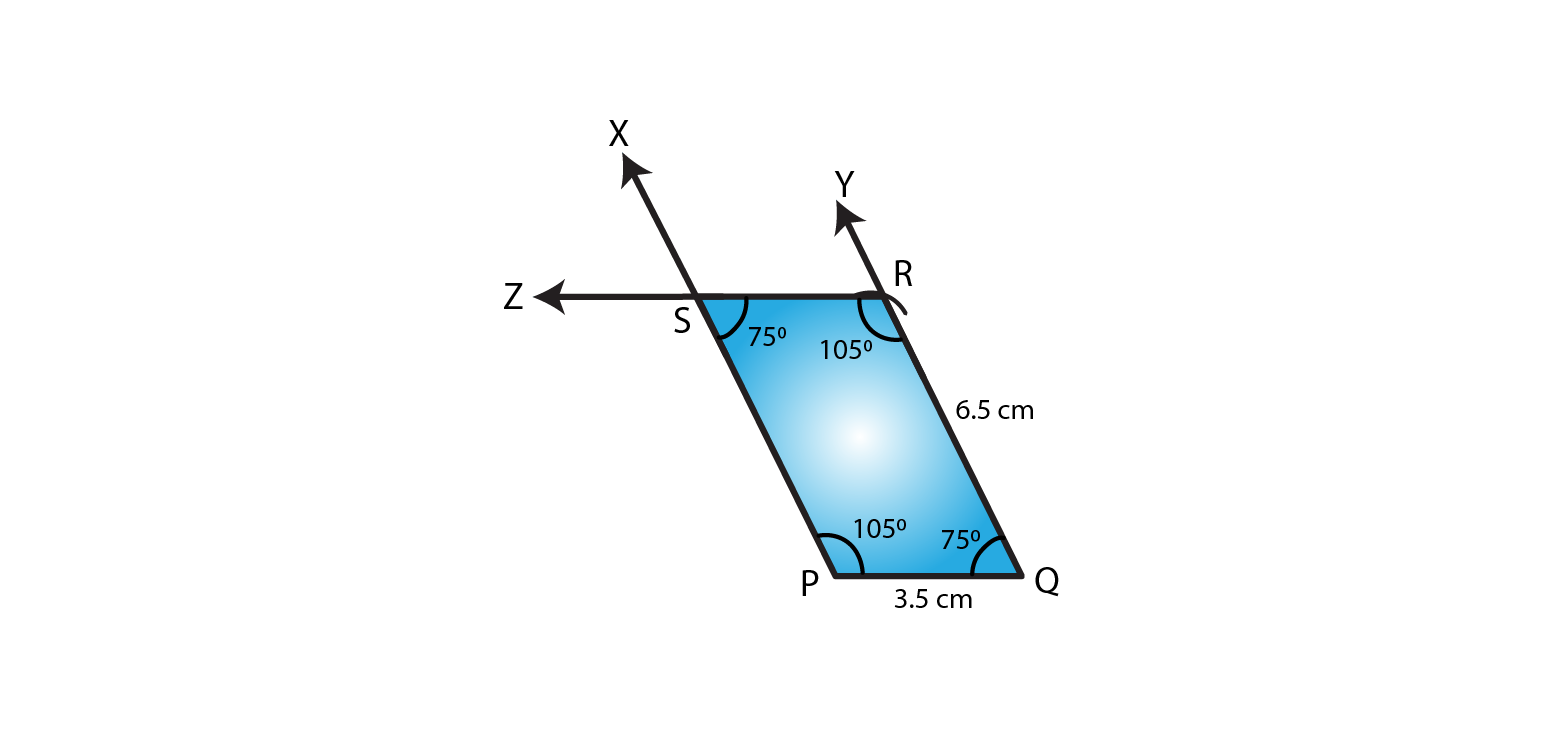4. Construct a quadrilateral ABCD when BC = 5.5 cm, CD = 4.1 cm, ∠A = 70°, ∠B = 110° and ∠D = 85°.

Solution:

The given details are BC = 5.5 cm, CD = 4.1 cm, ∠A = 70°, ∠B = 110° and ∠D = 85°.

We know that ∠A + ∠B + ∠C + ∠D = 360o

∴ ∠C = 95o

Step 1- Draw a line BC = 5.5cm

Step 2- Construct and angle of 110o at B.

Step 3- Construct and angle of 95o at C.

Step 4- Cut an arc of radius 4.1cm with C as the center to mark that point as D.

Step 5- Construct and angle of 85o at D such that it meets the line segment BX, mark that point as A.

Step 6- Now join CD, DA and BA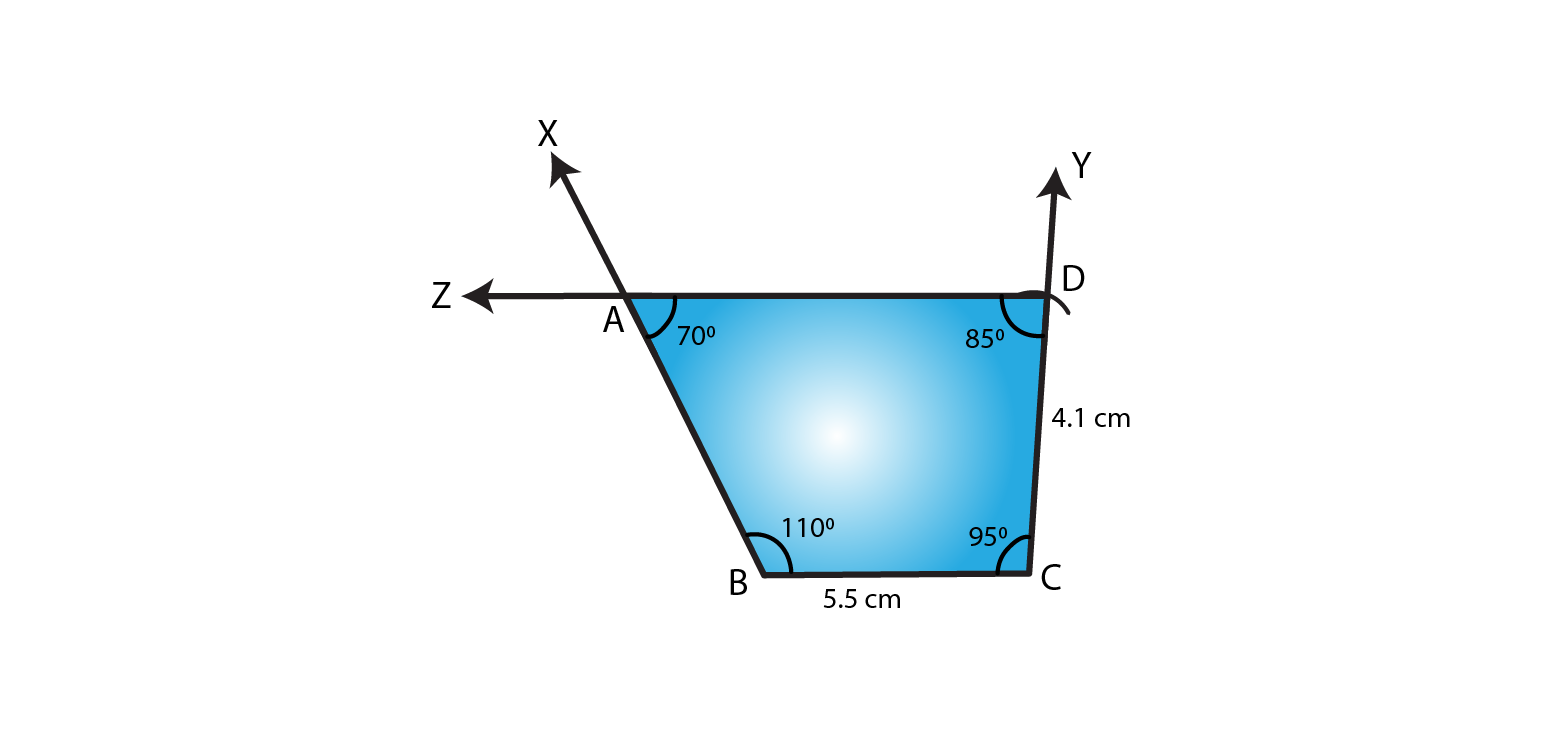5. Construct a quadrilateral ABCD ∠A = 65°, ∠B = 105°, ∠C = 75°, BC = 5.7 cm and CD = 6.8 cm.

Solution:

The given details are ∠A = 65°, ∠B = 105°, ∠C = 75°, BC = 5.7 cm and CD = 6.8 cm.

We know that ∠A + ∠B + ∠C + ∠D = 360o

∴ ∠D = 115o

Step 1- Draw a line BC = 5.7cm

Step 2- Construct and angle of 105o at B.

Step 3- Construct and angle of 75o at C.

Step 4- Cut an arc of radius 6.8cm with C as the center to mark that point as D.

Step 5- Construct and angle of 115o at D such that it meets the line segment BX, mark that point as A.

Step 6- Now join CD, DA and BA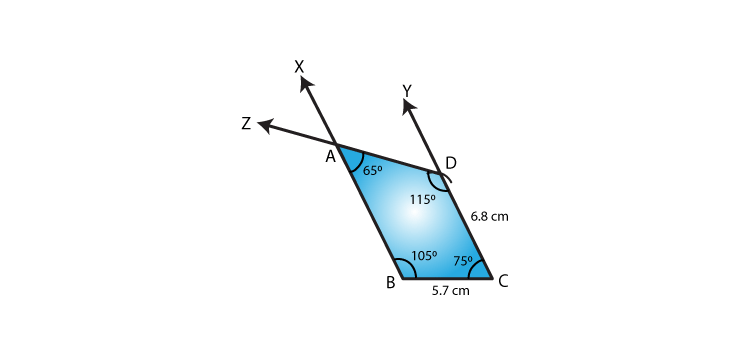6. Construct a quadrilateral PQRS in which PQ = 4 cm, QR = 5 cm ∠P = 50°, ∠Q = 110° and ∠R = 70°.

Solution:

The given details are PQ = 4 cm, QR = 5 cm ∠P = 50°, ∠Q = 110° and ∠R = 70°.

Step 1- Draw a line PQ = 4cm

Step 2- Construct and angle of 50o at P.

Step 3- Construct and angle of 110o at Q.

Step 4- Cut an arc of radius 5cm with Q as the center to mark that point as R.

Step 5- Construct and angle of 70o at R such that it meets the line segment PX, mark that point as S.

Step 6- Now join QR, RS and PS